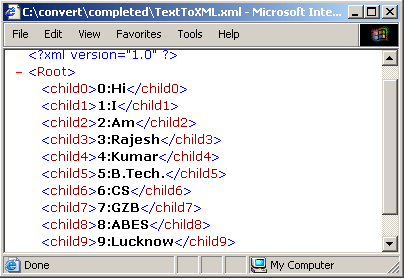Tutorials

# Convert Text To XML

Here we are discussing the conversion of a text file into xml file by giving an example. This example creates an xml file with its root node and child node. Then pass values into the child node. These values are in text format which is taken as input from

Here we are discussing the conversion of a text file into xml file by giving an example. This example creates an xml file with its root node and child node. Then pass values into the child node. These values are in text format which is taken as input from

# Convert Text To XML

Here we are discussing the conversion of a text file into xml file by giving an example. This example creates an xml file with its root node and child node. Then pass values into the child node. These values are in text format which is taken as input from console.

To convert text into xml file first create a xml file by using FileInputStream.  Modify the xml file by using OutputStreamReader. Use BufferedReader to take the input from console. In this example we are taking 10 string  from console and store these strings into an array of string. First write xml version tag e.g.<?xml version=\"1.0\"?> then create the root and child node into xml file. Pass  array string as value of nodes. We are inserting  ten nodes and passing the values at each nodes into xml file.

Here is the code of the program :

 ``` import java.math.*; import java.io.*; public class  TextToXML{  public static void main(String arg[]){   try{   String text[]=new String;   FileOutputStream fout = new FileOutputStream ("TextToXML.xml");   OutputStreamWriter out = new OutputStreamWriter (fout);     InputStreamReader in= new InputStreamReader (System.in);   BufferedReader bin= new BufferedReader(in);   for(int i=0;i<10;i++)   text[i]=bin.readLine();     out.write("\r\n");     out.write("\r\n");     for (int i = 0; i < 10; i++){   out.write("  "+i+":"+text[i]+ "");   }   out.write("");   out.close();   }catch (IOException e){   System.err.println(e);   }   } } ```

Input on command prompts:

 `C:\convert\completed>javac TextToXML.java` ```C:\convert\completed>java TextToXML Hi I Am Rajesh Kumar B.Tech. CS GZB ABES Lucknow```

Output of the example :`Download this example.`